Second Law Of Thermodynamics MCQ Level – 2 (Part - 1)

# Second Law Of Thermodynamics MCQ Level – 2 (Part - 1)

Test Description

## 10 Questions MCQ Test Topic wise Tests for IIT JAM Physics | Second Law Of Thermodynamics MCQ Level – 2 (Part - 1)

Second Law Of Thermodynamics MCQ Level – 2 (Part - 1) for Physics 2023 is part of Topic wise Tests for IIT JAM Physics preparation. The Second Law Of Thermodynamics MCQ Level – 2 (Part - 1) questions and answers have been prepared according to the Physics exam syllabus.The Second Law Of Thermodynamics MCQ Level – 2 (Part - 1) MCQs are made for Physics 2023 Exam. Find important definitions, questions, notes, meanings, examples, exercises, MCQs and online tests for Second Law Of Thermodynamics MCQ Level – 2 (Part - 1) below.
Solutions of Second Law Of Thermodynamics MCQ Level – 2 (Part - 1) questions in English are available as part of our Topic wise Tests for IIT JAM Physics for Physics & Second Law Of Thermodynamics MCQ Level – 2 (Part - 1) solutions in Hindi for Topic wise Tests for IIT JAM Physics course. Download more important topics, notes, lectures and mock test series for Physics Exam by signing up for free. Attempt Second Law Of Thermodynamics MCQ Level – 2 (Part - 1) | 10 questions in 45 minutes | Mock test for Physics preparation | Free important questions MCQ to study Topic wise Tests for IIT JAM Physics for Physics Exam | Download free PDF with solutions
 1 Crore+ students have signed up on EduRev. Have you?
Second Law Of Thermodynamics MCQ Level – 2 (Part - 1) - Question 1

### The property of a working substance which increases or decreases as the heat is supplied or removed in a reversible manner is known as. Select one:

Detailed Solution for Second Law Of Thermodynamics MCQ Level – 2 (Part - 1) - Question 1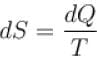Hence, entropy increases or decreases as heat is supplied or removed in reversible manner.

Second Law Of Thermodynamics MCQ Level – 2 (Part - 1) - Question 2

### An ideal gas has temperature T1 at the initial state i shown in the p-V diagram here. The gas has a higher temperature T2 at final states a and b, which it can reach along the path shown. The entropy change along the path to state a is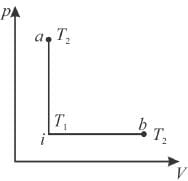Select one:

Detailed Solution for Second Law Of Thermodynamics MCQ Level – 2 (Part - 1) - Question 2

From i to a and i to b, change in temperature is same, hence dU remains same for both the processes. But i to a is isochoric process, hence work done is zero.
⇒ heat gained in process a is smaller, hence the entropy change along the pathto state a is smaller than b.
The correct answer is: smaller than b

Second Law Of Thermodynamics MCQ Level – 2 (Part - 1) - Question 3

### The T-S diagram of two cycles for the operation of an engine are shown in figure below.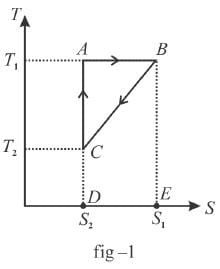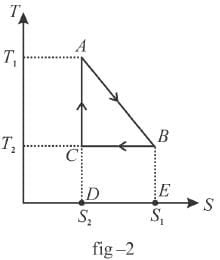The numerical values of the parameters T1 , T2 , S1 and S2 in the two figures are the same. Which cycle has greater efficiency? Select one:

Detailed Solution for Second Law Of Thermodynamics MCQ Level – 2 (Part - 1) - Question 3

Efficiency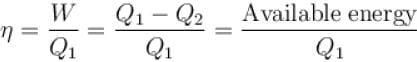Available energy per cycle is = Q1 – Q2
= Area of curve ABC
Q1 = Total area of curve (ABCD)
⇒ Area of curve is same for both figures, but total area is more for figure 1.
⇒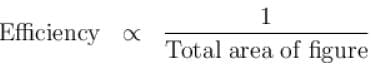Hence, second figure, has greater efficiency

Second Law Of Thermodynamics MCQ Level – 2 (Part - 1) - Question 4

The difference in entropy between a state of volume Vi and a state of volume of Vf (temperature and number of molecules remaining constant) is equal to.
Select one:

Detailed Solution for Second Law Of Thermodynamics MCQ Level – 2 (Part - 1) - Question 4

dS =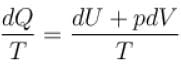for constant temperature, dU = 0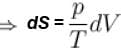and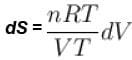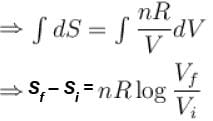The correct answer is: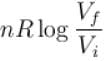Second Law Of Thermodynamics MCQ Level – 2 (Part - 1) - Question 5

The efficiency of the Carnot cycle may be increased by.
Select one:

Detailed Solution for Second Law Of Thermodynamics MCQ Level – 2 (Part - 1) - Question 5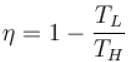if TL is reduced, η can be increased.
The correct answer is: decreasing the lowest temperature

Second Law Of Thermodynamics MCQ Level – 2 (Part - 1) - Question 6

Kelvin-Plank’s law deals with
Select one:

Detailed Solution for Second Law Of Thermodynamics MCQ Level – 2 (Part - 1) - Question 6

Kelvin-Plank statement of the second law of thermodynamics states that it is impossible to devise a cyclically operating device, the sole effect of which is to absorb energy in the form of heat from a single thermal reservoir and to deliver an equivalent amount of work.
The correct answer is: conversion of heat into work

Second Law Of Thermodynamics MCQ Level – 2 (Part - 1) - Question 7

In a reversible process, entropy of the system.
Select one:

Detailed Solution for Second Law Of Thermodynamics MCQ Level – 2 (Part - 1) - Question 7

For all reversible processes and constitutes part of the second law of thermodynamics, the entropy of the universe remains constant in a reversible process.

Second Law Of Thermodynamics MCQ Level – 2 (Part - 1) - Question 8

1gm of perfect gas at volume VA , pressure pA and temperature TA changes from state A to state B when volume is VB, pressure is pB and temperature TB. the change in entropy is.
Select one:

Detailed Solution for Second Law Of Thermodynamics MCQ Level – 2 (Part - 1) - Question 8

Change in entropy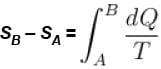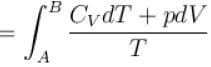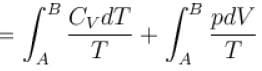pV = RT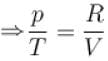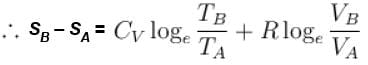The correct answer is: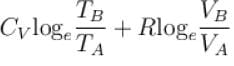Second Law Of Thermodynamics MCQ Level – 2 (Part - 1) - Question 9

Change in entropy depends
Select one:

Detailed Solution for Second Law Of Thermodynamics MCQ Level – 2 (Part - 1) - Question 9

Entropy is a measure of the number of microscopic configurations that a thermodynamic system can have when in a state as specified by some macroscopic variable.
The correct answer is: on the thermodynamic state

Second Law Of Thermodynamics MCQ Level – 2 (Part - 1) - Question 10

In an irreversible process, the entropy of the universe.
Select one:

Detailed Solution for Second Law Of Thermodynamics MCQ Level – 2 (Part - 1) - Question 10

When dealing with a system that is not isolated from its surroundings, remember that the increase in entropy described in the second law is that of the system and its surroundings. When a system and its surrounding interact in an irreversible process, the increase in entropy of one is greater than the decrease in entropy of the other. Hence, we conclude that the change in entropy of the universe must be greater than zero for an irreversible process and equal to zero for a reversible process.

## Topic wise Tests for IIT JAM Physics

217 tests
 Use Code STAYHOME200 and get INR 200 additional OFF Use Coupon Code
Information about Second Law Of Thermodynamics MCQ Level – 2 (Part - 1) Page
In this test you can find the Exam questions for Second Law Of Thermodynamics MCQ Level – 2 (Part - 1) solved & explained in the simplest way possible. Besides giving Questions and answers for Second Law Of Thermodynamics MCQ Level – 2 (Part - 1), EduRev gives you an ample number of Online tests for practice

217 tests